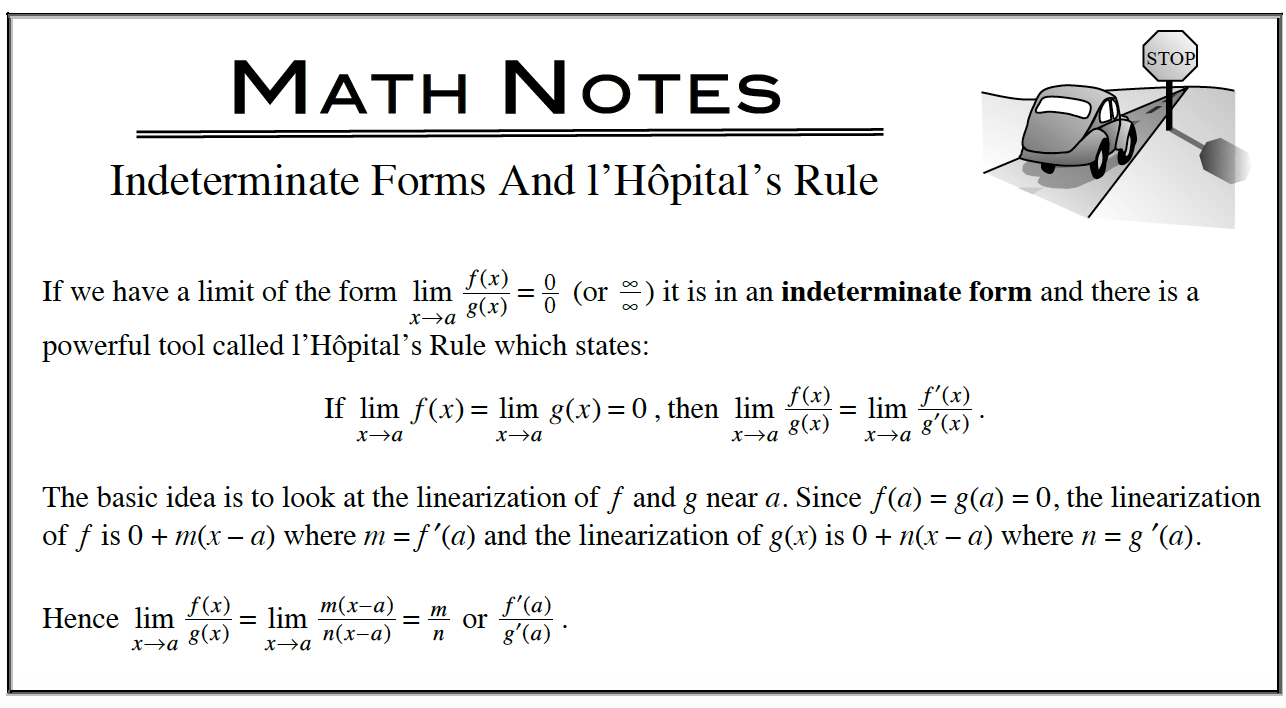### Home > APCALC > Chapter 5 > Lesson 5.5.2 > Problem5-174

5-174.

Use the definition of derivative as a limit to write an expression representing each of the following derivatives. Then evaluate each expression to write the actual derivative. l’Hôpital’s Rule may be used. Homework Help ✎

1. $f ′(x)$, where $f(x)=\frac { 1 } { 2 x + 1 }$

$f'(x)=\lim_{h\rightarrow 0}\frac{f(x+h)-f(x)}{h}$

Setup the definition of the derivative as a limit.
This is Hana's Method:

$f'(x)=\lim_{h\rightarrow 0}\frac{\frac{1}{2(x+h)+1}-\frac{1}{2x+1}}{h}$Make a choice about how to evaluate this limit. These are your choices:
1) Use algebra. Simplify the fraction until you can 'cancel out' the h in the denominator and then evaluate as h→0.
2) Deconstruct Hana's Method. Once you identify f(x), find f '(x) using the Power Rule. Of course, in this case, that is working in circles.
3) Use l'Hôpital's Rule; after all, there is a 0 in the numerator and the denominator.

2. $h′(x)$, where $h(x) = (x - 2)^{-2}$

Refer to the hints in part (a).

3. $g′(4)$, where $g(x)=\sqrt { x }$

Refer to the hints in part (a).